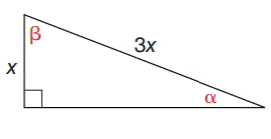Chapter 11.1, Problem 25EElementary Geometry For College St...

7th Edition
Alexander + 2 others
ISBN: 9781337614085

Solutions

Chapter
SectionElementary Geometry For College St...

7th Edition
Alexander + 2 others
ISBN: 9781337614085
Textbook Problem

In Exercises 21 to 26, find the measures of the angles named to the nearest degree.To determine

To measure:

The angle named to the nearest degree.

Explanation

In a right angle triangle,

1) The side PQ, which is opposite to the right angle PRQ is called the hypotenuse.

(The hypotenuse is the longest side of the right triangle.)

2) The side RQ is called the adjacent side of angle θ.

3) The side PR is called the opposite side of angle θ.

Formula used:

sinθ=oppositehypotenuse

Given:

By using Pythagoras theorem, that is

c2=a2+b2

Let b be the adjacent side of the triangle.

From, the diagram we have c=3x and a=x

(3x)2=x2+b29x2=x2+b2b2=9x2x2b2=8x2b=8x2b=2.83x

For angle α, the opposite side is x and hypotenuse side is 3x

Still sussing out bartleby?

Check out a sample textbook solution.

See a sample solution

The Solution to Your Study Problems

Bartleby provides explanations to thousands of textbook problems written by our experts, many with advanced degrees!

Get Started

In Exercises 1124, find the indicated limits, if they exist. 16. limx2x22x3x2+5+6

Applied Calculus for the Managerial, Life, and Social Sciences: A Brief Approach

Define the terms population and sample, and explain the role of each in a research study.

Essentials of Statistics for The Behavioral Sciences (MindTap Course List)

Describe the scores in a sample that has standard deviation of zero.

Statistics for The Behavioral Sciences (MindTap Course List)

True or False: The Alternating Series Test may be applied to determine the convergence of n=12+(1)n2n2.

Study Guide for Stewart's Single Variable Calculus: Early Transcendentals, 8th

For and , a × b =

Study Guide for Stewart's Multivariable Calculus, 8th